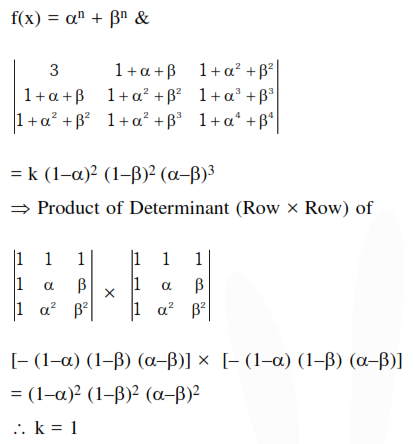# Solve this following Question

Question:

If $\alpha, \beta \neq 0$, and $f(\mathrm{n})=\alpha^{\mathrm{n}}+\beta^{\mathrm{n}}$ and $\left|\begin{array}{ccc}3 & 1+f(1) & 1+f(2) \\ 1+f(1) & 1+f(2) & 1+f(3) \\ 1+f(2) & 1+f(3) & 1+f(4)\end{array}\right|=\mathrm{K}(1-\alpha)^{2}(1-\beta)^{2}(\alpha-\beta)^{2}$ then $\mathrm{K}$ is equal to :

1. $\alpha \beta$

2. $\frac{1}{\alpha \beta}$

3. 1

4. -1

Correct Option: , 3

Solution: